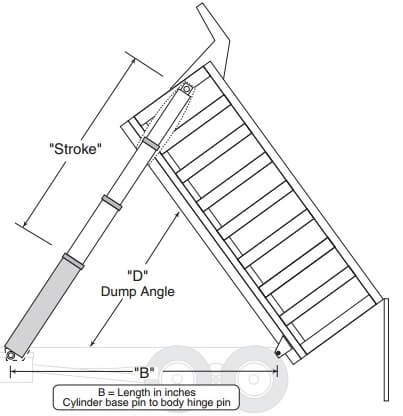# What hydraulic cylinder do I need for my dump body?

November 1, 2016

Measuring the hydraulic cylinder lift requirements for a front mount, frame type, dump body might often seem like guesswork, but it doesn’t have to be. Through a few simple calculations we can accurately determine the requirements needed to lift our fully loaded dump body, and the stroke needed to get it there. These calculations won’t work however, for a frameless trailer or twin under body hoist. We’ll cover those in a future article.First though, we’ll need to get some measurements. We need to determine exactly where the hinge of the dump body is, and then measure from that point, forward to the center of the load. This is going to generally be the middle of the dump body. Be sure to take that measurement in inches.

From that same hinge location, measure forward to where the cylinder connects to the dump body. This measurement will be larger than the previous, and should be in inches as well. This is our cylinder base point. With these two numbers in hand, we just need to get the weight of the load we are lifting, and we are ready to do some calculations.Because the laws of physics work in our favor, the initial hydraulic cylinder force will be the highest. As the hydraulic cylinder extends, raising the dump body, the force will decrease as the dump body continues to rise. To get the initial force required for lifting, take the load in pounds, and multiply it by the first number (distance from hinge to center of load). Divide the new number by the distance from the hinge to the lifting point, and we now have our initial force required to lift the load. (Example: 50,000lbs of load with 85” to center of load, and 166” to cylinder base point would require a cylinder capable of lifting 25,603lbs of force to get the lift started.Now that we know the lifting force required, we can determine the stroke needed based on our dump body. We’ll need that number in inches again from the hinge to the hydraulic cylinder base point on the dump body. We then a “multiplier” to use to get the required stroke. The multiplier is a number based on the dump angle. A normal dump angle ranges from 45-57 degrees. Use the chart at the left to find the correct multiplier. Now multiply the base length in inches with the multiplier and we get the stroke. (Example: 166” cylinder base point measurement times our multiplier of .845 (50 degrees) gives us a stroke length of 140”)

With these two numbers in hand, there’s only one step left, and that’s to call the hydraulic cylinder experts at Hydraulic Cylinders Inc to find the best hydraulic cylinder for your front mount, frame type dump body needs at (888)771-1894.